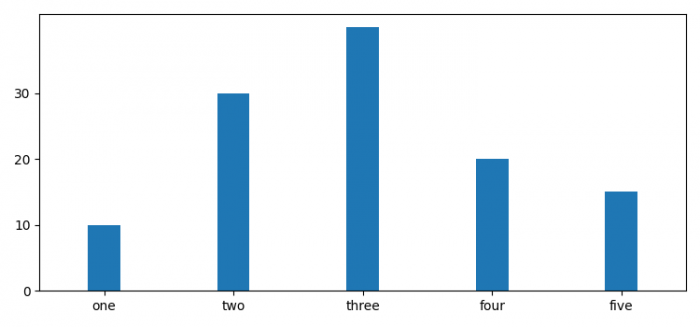# How to put gap between Y-axis and the first bar in a vertical barchart in Matplotlib?

MatplotlibPythonData Visualization

#### Python Data Science basics with Numpy, Pandas and Matplotlib

Most Popular

63 Lectures 6 hours

#### Data Visualization using MatPlotLib & Seaborn

11 Lectures 4 hours

#### MatPlotLib with Python

9 Lectures 2.5 hours

To put gap between Y-axis and the first bar in vertical barchart, we can reduce the X-axis scale.

## Steps

• Set the figure size and adjust the padding between and around the subplots.
• Create lists x_val, x_names andvaldata points. Also, initialize width and interval variables.
• Make a bar plot using bar() method.
• Get or set the current tick locations and labels of the X-axis.
• Get or set the current tick locations and labels of the Y-axis.
• Get or set the x limits of the current axes.
• To display the figure, use show() method.

## Example

from matplotlib import pyplot as plt

plt.rcParams["figure.figsize"] = [7.50, 3.50]
plt.rcParams["figure.autolayout"] = True

x_val = range(5)
x_names = ["one", "two", "three", "four", "five"]
y_val = [10, 30, 40, 20, 15]

width = 0.25
interval = 10

plt.bar(x_val, y_val, width=width, align='center')
plt.xticks(x_val, x_names)

plt.yticks(range(0, max(y_val), interval))
plt.xlim([min(x_val) - 0.5, max(x_val) + 0.5])

plt.show()

## Output